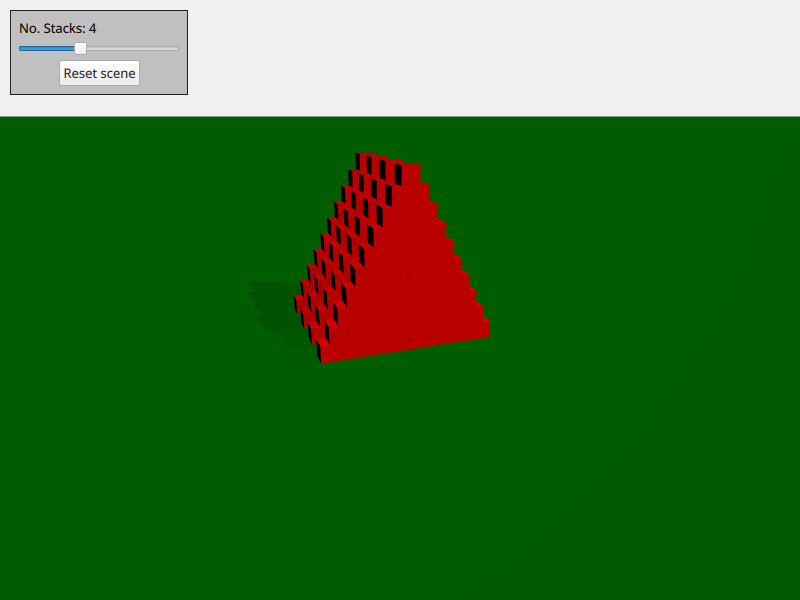# Qt Quick 3D Physics - Cannon Example

Demonstrates how to spawn physical objects.This example demonstrates how to create and delete physical objects on demand. The scene consists of a number of stacks of boxes. You can move around by using `WASD` and the mouse and shoot a ball by pressing `space`.

The scene is setup with the usual Qt Quick 3D objects like view, camera and light:

```PerspectiveCamera {
id: camera
position: Qt.vector3d(-4000, 5000, 10000)
eulerRotation: Qt.vector3d(-20, -20, 0)
clipFar: 500000
clipNear: 100
}
DirectionalLight {
eulerRotation: Qt.vector3d(-45, 45, 0)
brightness: 1
}```

We also add a static floor:

```StaticRigidBody {
eulerRotation: Qt.vector3d(-90, 0, 0)
collisionShapes: PlaneShape {}
Model {
source: "#Rectangle"
scale: Qt.vector3d(2000, 2000, 1)
materials: PrincipledMaterial {
baseColor: "green"
}
}
}```

We create a Node we use as the spawner of objects and put inside our view:

```Node {
id: shapeSpawner
property var instancesBoxes: []
property var instancesSpheres: []
property int stackCount: 0
property var boxComponent: Qt.createComponent("Box.qml")
property var sphereComponent: Qt.createComponent("Sphere.qml")

function createStack(stackZ, numStacks) {
let size = 10
let extents = 400

for (var i = 0; i < size; i++) {
for (var j = 0; j < size - i; j++) {
let x = j * 2 - size + i
let y = i * 2 + 1
let z = 5 * (stackZ - numStacks)
let center = Qt.vector3d(x, y, z).times(0.5 * extents)
let box = boxComponent.incubateObject(shapeSpawner, {
"position": center,
"xyzExtents": extents
})
instancesBoxes.push(box)
}
}
}

function createBall(position, forward) {
var diameter = 600
var speed = 20000
let settings = {
"position": position,
"sphereDiameter": diameter
}
let sphere = sphereComponent.createObject(shapeSpawner, settings)
sphere.setLinearVelocity(forward.times(speed))
instancesSpheres.push(sphere)

if (sphere === null) {
console.log("Error creating object")
}
}

function reset() {
// Only run method if previous stack has been created fully
for (var i = 0; i < instancesBoxes.length; i++)
if (!instancesBoxes[i].object)
return

instancesSpheres.forEach(sphere => {
sphere.collisionShapes = {}
sphere.destroy()
})
instancesBoxes.forEach(box => {
box.object.collisionShapes = {}
box.object.destroy()
})
instancesSpheres = []
instancesBoxes = []

for (var stackI = 0; stackI < stackSlider.value; stackI++) {
shapeSpawner.createStack(stackI, stackSlider.value)
}
}
}```

We have three methods: `createStack` for creating a stack, `createBall` for creating a ball with velocity and `reset` for resetting the scene. The actual box and sphere that is spawn is stored in their own qml files (`box.qml` and `sphere.qml`).

Files: